Next: Lateral connections in Up: Low-Level Vision Previous: Shaping the RFs

## Pairs of RFs

A connection between the mechanisms of low-level vision, as exemplified by the RFs of the V1 neurons, and the demands of higher-level tasks such as object recognition, is provided by the recent psychophysical results concerning the dependence of recognition performance on the viewpoint (see  for a review), and their implications regarding the nature of representation of 3D shapes. For example, in one series of studies, psychophysical findings of viewpoint-dependent performance of human subjects in the recognition of computer-generated wireframe 3D shapes [4,11] were accompanied by a model of recognition, in which each 3D object known to the system was represented by a collection of its 2D views [4,10].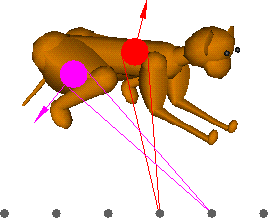Figure 3: A 3D object and a pair of RFs. The output of a bank of RFs that process images of an object undergoing rotation in 3D changes with viewpoint. To increase the invariance of RF-based representations in the face of such changes, RFs may be paired according to a criterion described in the Pairs of RFs section; this pairing may be supported by lateral connections.

The applicability of the particular view-based model described in  to stimuli other than wireframe objects is limited because it encodes shapes by the coordinates of the wire's vertices. A natural way to extend that model to deal with realistic shapes is to provide it with a preprocessing stage consisting of a bank of receptive fields which would transduce the input images into points in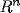(see Figure 3). As argued in , the criterion for the choice of RFs in that case would be faithful representation of similarity: points corresponding to views that belong to the same object should be situated closer to each other than points corresponding to views of different objects (cf. ). In other words, the task of a model that involves view-based representations can be facilitated by making the similarity relationships in input space reflect as closely as possible the true similarities between objects prevailing in the world, even when complete invariance with respect to viewpoint is unattainable.

The rest of this section shows how to construct an RF-based representation that is relatively stable under consistent changes in the object's attitude with respect to the observer (see  for details). Consider a rigid object undergoing rotation around an arbitrary but fixed axis in depth. Pick at random two patches,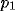and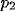, on the object's surface, and let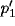and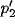be the corresponding patches after a small rotation around a fixed axis. Assume that there is a distant point light source in the direction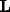, that the object's surface is Lambertian, and that the mean albedo atandis, respectively,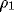and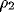. Then the intensities at the two patches before rotation are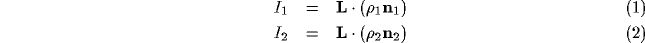where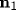and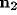are the surface normals atand. Following the rotation, the intensities are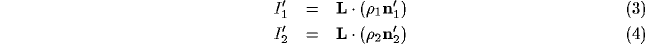where the assumption of a distant light source was used to equate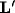with. Taking the difference between intensities of the two patches, one obtains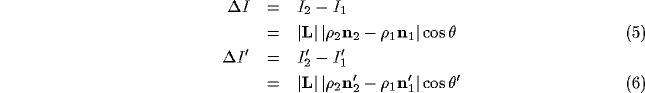where(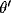) is the angle betweenand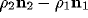before (after) the rotation. Because the object was assumed rigid, we have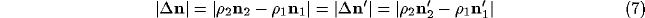This means that the magnitude of the vectorthat expresses the difference of orientation between patchesandis invariant under the rotation. Thus, if the quantity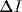changes following rotation (that is, if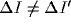), this could be only due to a change in the orientation of the vectorwith respect to the direction of the illumination.

In the special case when the vectoris parallel to the axis of rotation, the anglewill not change, and, consequently, the difference in intensity between the two patches,, will remain invariant under rotation. Consider now a set of locally averaged measurements of intensity such as the one provided by the set of receptive fields in Figure 3. To obtain an invariant representation of an object by a subset of those measurements, one should pick pairs of RFs for which the difference in activity is stable over small rotations of the object. For any such pair of RFs, and for a fixed axis of rotation,will then remain stable. A snapshot of activities of the chosen set of RF pairs can be used to represent the object (for a different object, another set of RF pairs will have to be picked). As suggested in , the pairing of RFs necessary for obtaining invariance under the specified conditions can be supported by lateral connections.Next: Lateral connections in Up: Low-Level Vision Previous: Shaping the RFs